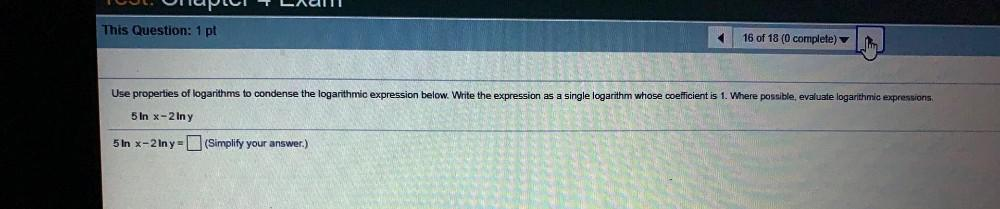Question:

# Tel. nupul Ladu This Question: 1 pt 16 of 18 (0 complete) Use properties of logarithms to condense the logarithmic expression beTel. nupul Ladu This Question: 1 pt 16 of 18 (0 complete) Use properties of logarithms to condense the logarithmic expression below. Write the expression as a single logarithm whose coefficient is 1. Where possible, evaluate logarithmic expressions. 5 In x-2 In y 5 ln x-2 Iny = (Simplify your answer.)